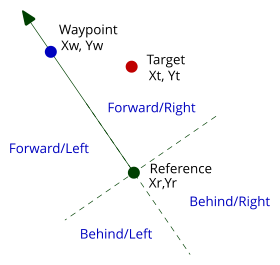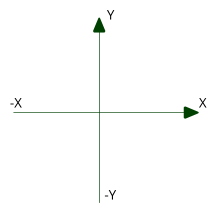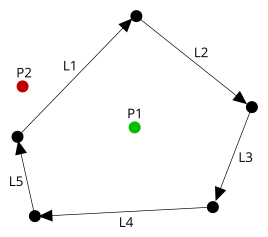# Applications of dot and cross products

Sometimes in graphical applications there is a need to know the relative position of a point with respect to a line from a reference point.Where is the target in relation to the reference and its direction?
Dot and cross products can make this an easy task.

Let’s start with a reminder of what dot and cross products are.

Take two vectors x, y1 and x, y2

The dot product is (x1 * x2) + (y1 * y2and the cross product is (x1 * y2) – (x2 * y1)Take the example above. We have a reference object at Xr,Yr facing in the direction of the arrow.
The target is at point Xt,Yt
A arbitrary point on the line from the reference in the specified direction is Xw,Yw

First, find the relative coordinates of the target and waypoint relative to the reference.

dXw = Xw – Xr
dYw = Yw – Yr
dXt = Xt – Xr
dYt = Yt – Yr

Now find the dot and cross products of these vectors.

Dot = (dXw * dXt) + (dYw * dYt)
Cross = (dXw * dYt) – (dXt * dYw)

Assuming the coordinate system is as below.Dot > 0, Cross < 0 : The target is forward of the reference and to the right.

Dot > 0, Cross > 0 : The target is forward of the reference and to the left.

Dot < 0, Cross < 0 : The target is behind the reference and to the right.

Dot < 0, Cross > 0 : The target is behind the reference and to the left.

‘Forward’, ‘Behind’, ‘Left’ and ‘Right’ are relative to the reference’s direction. This calculation will be correct for any direction.

Other uses

This technique can also be used to determine if a point is within a closed convex hull.A closed convex hull defined by the lines L1, L2, L3, L4 L5.
Point P1 is inside the hull, point P2 is outside.

For P1, the cross product for all of the lines will indicate that the it is to the right and therefore, must be inside.
For P2, the cross product for L1 will put it on the left hand side and therefore it cannot be inside the hull.

Why does this work?

The dot product of vectors A and B is |A||B|cos(Ø)
The cross product of vectors A and B is |A||B|sin(Ø)
Where Ø is the angle between the vectors A and B. Range +-180°

Therefore the sign of the result indicates the quadrant that target occupies in the circle around the reference, relative to the reference’s direction.